JMSLTM Numerical Library 7.2.0
com.imsl.math

## Class SparseCholesky

• All Implemented Interfaces:
Serializable

```public class SparseCholesky
extends Object
implements Serializable```
Sparse Cholesky factorization of a matrix of type `SparseMatrix`.

Class `SparseCholesky` computes the Cholesky factorization of a sparse symmetric positive definite matrix A. This factorization can then be used to compute the solution of the linear system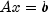.

Typically, the solution of a large sparse positive definite systemis done in 4 steps:

1. In step one, an ordering algorithm is used to preserve sparsity in the Cholesky factor L of matrix A during the numerical factorization process. The new order can be described by a permutation matrix P.
2. Step two consists of setting up the data structure for the Cholesky factor L, where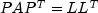. This step is called the symbolic factorization phase of the computation. During symbolic factorization, only the sparsity pattern of sparse matrix A, i.e., the locations of the nonzero entries of matrix A are needed but not any of the elements themselves.
3. In step 3, the numerical factorization phase, the Cholesky factorization is done numerically.
4. Step 4 is the solution phase. Here, the numerical solution, x, to the original system is obtained by solving the two triangular systems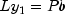,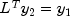and the permutation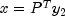.

Class `SparseCholesky` realizes all four steps by algorithms described in George and Liu (1981). Especially, step one, is a realization of a minimum degree ordering algorithm. The numerical factorization in its standard form is based on a sparse compressed storage scheme. Alternatively, a multifrontal method can be used. The multifrontal method requires more storage but will be faster than the standard method in certain cases. The multifrontal method is based on the routines in Liu (1987). For a detailed description of this method, see Liu (1990), also Duff and Reid(1983, 1984), Ashcraft (1987) et al. (1987), and Liu (1986, 1989, 1992). The numerical factorization method can be specified by using the ``` setNumericFactorizationMethod```.

The `solve` method will compute the symbolic and numeric factorizations if they have not already been computed or supplied by the user through the `factorSymbolically`, `factorNumerically`, `setNumericFactor`, or `setSymbolicFactor` methods. These factorizations are retained for later use by the `solve` method when different right-hand sides are to be solved.

There is a special situation where computations can be simplified. If an application generates different sparse symmetric positive definite coefficient matrices that all have the same sparsity pattern, then by using methods `getSymbolicFactor` and `setSymbolicFactor` the symbolic factorization needs only be computed once.

Example, Serialized Form
• ### Nested Class Summary

Nested Classes
Modifier and Type Class and Description
`static class ` `SparseCholesky.NotSPDException`
The matrix is not symmetric, positive definite.
`static class ` `SparseCholesky.NumericFactor`
The numeric Cholesky factorization of a matrix.
`static class ` `SparseCholesky.SymbolicFactor`
The symbolic Cholesky factorization of a matrix.
• ### Field Summary

Fields
Modifier and Type Field and Description
`static int` `MULTIFRONTAL_METHOD`
Indicates the multifrontal method will be used for numeric factorization.
`static int` `STANDARD_METHOD`
Indicates the method of George/Liu (1981) will be used for numeric factorization.
• ### Constructor Summary

Constructors
Constructor and Description
`SparseCholesky(SparseMatrix A)`
Constructs the matrix structure for the Cholesky factorization of a sparse symmetric positive definite matrix of type `SparseMatrix`.
• ### Method Summary

Methods
Modifier and Type Method and Description
`void` `factorNumerically()`
Computes the numeric factorization of a sparse real symmetric positive definite matrix.
`void` `factorSymbolically()`
Computes the symbolic factorization of a sparse real symmetric positive definite matrix.
`double` `getLargestDiagonalElement()`
Returns the largest diagonal element of the Cholesky factor.
`long` `getNumberOfNonzeros()`
Returns the number of nonzeros in the Cholesky factor.
`SparseCholesky.NumericFactor` `getNumericFactor()`
Returns the numeric Cholesky factor.
`int` `getNumericFactorizationMethod()`
Returns the method used in the numerical factorization of the permuted input matrix.
`double` `getSmallestDiagonalElement()`
Returns the smallest diagonal element of the Cholesky factor.
`SparseCholesky.SymbolicFactor` `getSymbolicFactor()`
Returns the symbolic Cholesky factor.
`void` `setNumericFactor(SparseCholesky.NumericFactor numericFactor)`
Sets the numeric Cholesky factor to use in solving of a sparse positive definite system of linear equations.
`void` `setNumericFactorizationMethod(int method)`
Defines the method used in the numerical factorization of the permuted input matrix.
`void` `setSymbolicFactor(SparseCholesky.SymbolicFactor symbolicFactor)`
Sets the symbolic Cholesky factor to use in solving a sparse positive definite system of linear equations.
`double[]` `solve(double[] b)`
Computes the solution of a sparse real symmetric positive definite system of linear equations.
• ### Methods inherited from class java.lang.Object

`clone, equals, finalize, getClass, hashCode, notify, notifyAll, toString, wait, wait, wait`
• ### Field Detail

• #### MULTIFRONTAL_METHOD

`public static final int MULTIFRONTAL_METHOD`
Indicates the multifrontal method will be used for numeric factorization.
Constant Field Values
• #### STANDARD_METHOD

`public static final int STANDARD_METHOD`
Indicates the method of George/Liu (1981) will be used for numeric factorization.
Constant Field Values
• ### Constructor Detail

• #### SparseCholesky

`public SparseCholesky(SparseMatrix A)`
Constructs the matrix structure for the Cholesky factorization of a sparse symmetric positive definite matrix of type `SparseMatrix`.
Parameters:
`A` - The `SparseMatrix` symmetric positive definite matrix to be factored. Only the lower triangular part of the input matrix is used.
• ### Method Detail

• #### factorNumerically

```public void factorNumerically()
throws SparseCholesky.NotSPDException```
Computes the numeric factorization of a sparse real symmetric positive definite matrix.

This method numerically factors the instance of the constructed matrix A, where A is of type `SparseMatrix` and is symmetric positive definite. The factorization is obtained in several steps:

1. First, matrix A is permuted to reduce fill-in, leading to a sparse symmetric positive definite matrix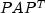.
2. Then, matrixis symbolically and numerically factored.

Note that the symbolic factorization is not done if the symbolic factor has been supplied by the user through the `setSymbolicFactor` method.

Throws:
`SparseCholesky.NotSPDException` - is thrown if the input matrix is not symmetric, positive definite.
• #### factorSymbolically

```public void factorSymbolically()
throws SparseCholesky.NotSPDException```
Computes the symbolic factorization of a sparse real symmetric positive definite matrix.

This method symbolically factors the instance of the constructed matrix A, where A is of type `SparseMatrix` and is symmetric positive definite. The factorization is obtained in several steps:

1. First, matrix A is permuted to reduce fill-in, leading to a sparse symmetric positive definite system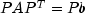.
2. Then, matrixis symbolically factored.

Throws:
`SparseCholesky.NotSPDException` - is thrown if the input matrix is not symmetric, positive definite.
• #### getLargestDiagonalElement

`public double getLargestDiagonalElement()`
Returns the largest diagonal element of the Cholesky factor.
Returns:
a `double` value specifying the largest diagonal element of the Cholesky factor. Use of this method is only sensible if a numeric factorization of the input matrix was done beforehand.
• #### getNumberOfNonzeros

`public long getNumberOfNonzeros()`
Returns the number of nonzeros in the Cholesky factor.
Returns:
a `long` value specifying the number of nonzeros (including the diagonal) of the Cholesky factor.
• #### getNumericFactor

`public SparseCholesky.NumericFactor getNumericFactor()`
Returns the numeric Cholesky factor.
Returns:
a `NumericFactor` containing the numeric Cholesky factor.
• #### getNumericFactorizationMethod

`public int getNumericFactorizationMethod()`
Returns the method used in the numerical factorization of the permuted input matrix.
Returns:
an `int` value equal to `STANDARD_METHOD` = 0 or `MULTIFRONTAL_METHOD` = 1 representing the method used in the numeric factorization of the permuted input matrix. See `setNumericFactorizationMethod` for more details.
• #### getSmallestDiagonalElement

`public double getSmallestDiagonalElement()`
Returns the smallest diagonal element of the Cholesky factor.
Returns:
a `double` value specifying the smallest diagonal element of the Cholesky factor. Use of this method is only sensible if a numeric factorization of the input matrix was done beforehand.
• #### getSymbolicFactor

`public SparseCholesky.SymbolicFactor getSymbolicFactor()`
Returns the symbolic Cholesky factor.
Returns:
a `SymbolicFactor` containing the symbolic Cholesky factor.
• #### setNumericFactor

`public void setNumericFactor(SparseCholesky.NumericFactor numericFactor)`
Sets the numeric Cholesky factor to use in solving of a sparse positive definite system of linear equations.
Parameters:
`numericFactor` - a `NumericFactor` object containing the numeric Cholesky factor. By default the numeric factorization is computed.
• #### setNumericFactorizationMethod

`public void setNumericFactorizationMethod(int method)`
Defines the method used in the numerical factorization of the permuted input matrix.
Parameters:
`method` - an `int` value specifying the method to choose:  `Method Name` Description `STANDARD_METHOD` standard method as described by George/Liu (1981). This is the default. `MULTIFRONTAL_METHOD` multifrontal method
Throws:
`IllegalArgumentException` - This exception is thrown when the value for method is not `STANDARD_METHOD` or `MULTIFRONTAL_METHOD`.
• #### setSymbolicFactor

`public void setSymbolicFactor(SparseCholesky.SymbolicFactor symbolicFactor)`
Sets the symbolic Cholesky factor to use in solving a sparse positive definite system of linear equations.
Parameters:
`symbolicFactor` - a `SymbolicFactor` containing the symbolic Cholesky factor. By default the symbolic factorization is computed.
• #### solve

```public double[] solve(double[] b)
throws SparseCholesky.NotSPDException```
Computes the solution of a sparse real symmetric positive definite system of linear equations.

This method solves the linear system, where A is symmetric positive definite. The solution is obtained in several steps:

1. First, matrix A is permuted to reduce fill-in, leading to a sparse symmetric positive definite system.
2. Then, matrixis symbolically and numerically factored.
3. The final solution is obtained by solving the systems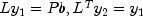and.

By default this method implements all of the above steps. The factorizations are retained for later use by subsequent solves. By choosing appropriate methods within this class, the computation can be reduced to the solution of the systemfor a given or precomputed symbolic or numeric factor.

Parameters:
`b` - a `double` vector of length equal to the order of matrix `A` representing the new right-hand side.
Returns:
a `double` vector of length equal to the order of matrix `A` representing the solution to the system of linear equations.
Throws:
`SparseCholesky.NotSPDException` - is thrown if the input matrix is not symmetric, positive definite.
JMSLTM Numerical Library 7.2.0